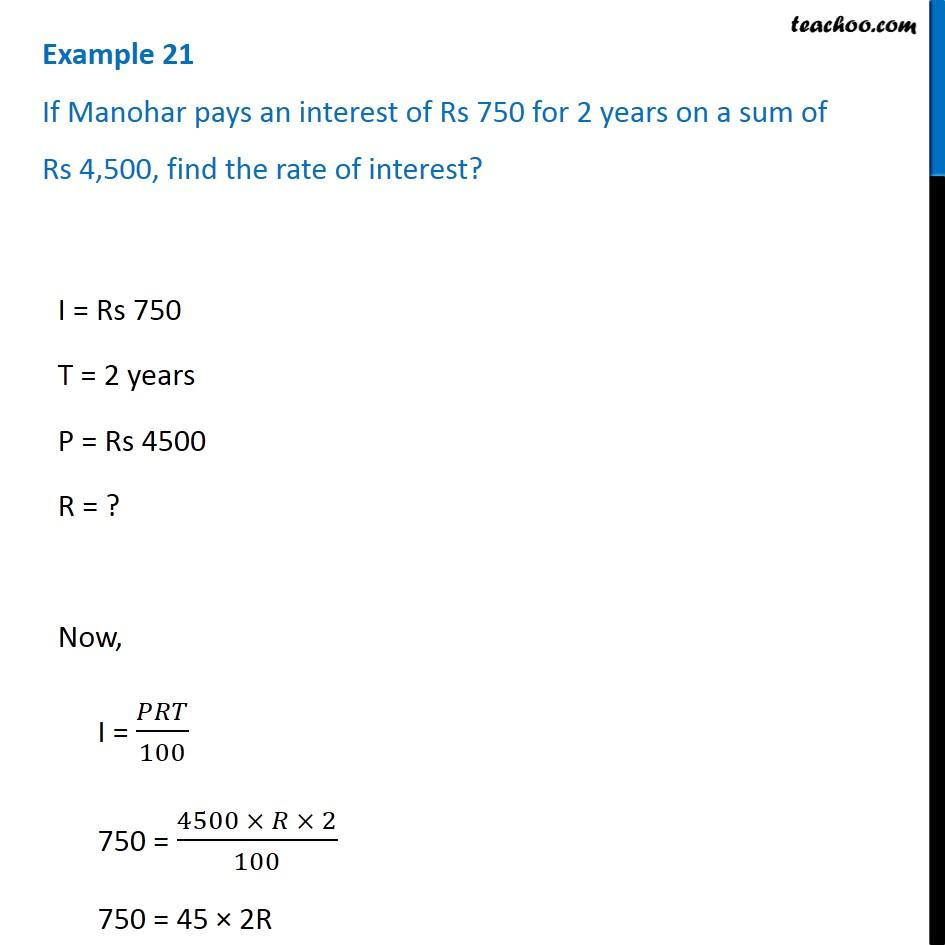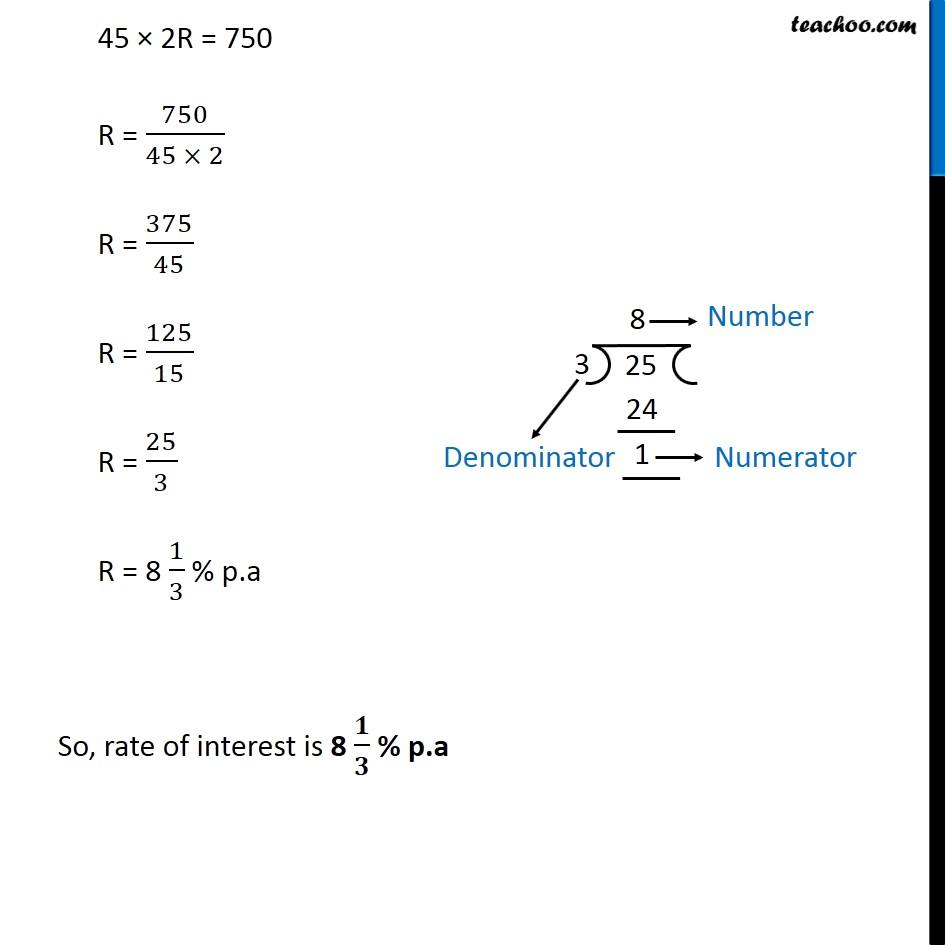Simple interest

Chapter 8 Class 7 Comparing Quantities
Concept wiseIntroducing your new favourite teacher - Teachoo Black, at only ₹83 per month

### Transcript

Example 21 If Manohar pays an interest of Rs 750 for 2 years on a sum of Rs 4,500, find the rate of interest? I = Rs 750 T = 2 years P = Rs 4500 R = ? Now, I = 𝑃𝑅𝑇/100 750 = (4500 × 𝑅 × 2)/100 750 = 45 × 2R 45 × 2R = 750 R = 750/(45 × 2) R = 375/45 R = 125/15 R = 25/3 R = 8 1/3 % p.a So, rate of interest is 8 𝟏/𝟑 % p.a